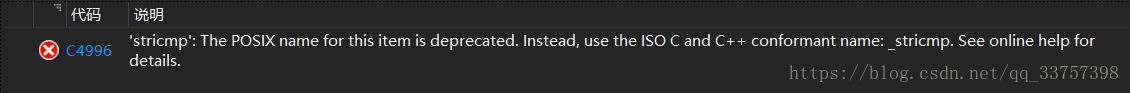# C语言字符串操作函数总结

## 1.strcpy函数

1）字符串str2会覆盖str1中的全部字符，

2）字符串str2的长度不能超过str1,

3)  拷贝原理：从首元素开始，遇到\0结束

int main(int argc, char const *argv[])
{
char *str1 = "hello world";
char *str2;

// 功能：把str1的内容拷贝到str2，参数为字符数组指针
strcpy(str2, str1);

printf("str2 = %s\n", str2);

char str3 = "hello\0 world";
char str4;

//拷贝原理：从首元素开始，遇到\0结束
strcpy(str4, str3);

printf("str4 = %s\n", str4);
return 0;
}

str2 = hello world
str4 = hello

## 2.strncpy函数

1）不会清除str1中全部字符串，只会改变前n个字符串，

2）n不能大于字符串str1、str2的长度

3）但是如果使用strncpy_s便会清除str1中的全部字符串

int main(int argc, char const *argv[])
{
char str1[] = "day day up";
char str2[] = "you are";
strncpy(str1, str2, strlen(str2));
printf("%s\n", str1); // you are up
return 0;
}

## 3.strcat函数

int main(int argc, char const *argv[])
{
char str1[] = "hello ";
char str2[] = "world";
strcat(str1, str2); // hello world
printf("%s\n", str1);
return 0;
}

## 4.strncat函数

int main(int argc, char const *argv[])
{
char str1[] = "hello ";
char str2[] = "world";
strncat(str1, str2, 2); // hello wo
printf("%s\n", str1);
return 0;
}

## 5.strlen函数

{
char *str1 = "hello world";
char *str2 = "hello,\0 kite";
int l1 = strlen(str1);
int l2 = strlen(str2);
printf("l1 = %d\n", l1); // 11
printf("l2 = %d\n", l2); // 6
return 0;
}

## 6.strcmp函数返回：0或一个正数或一个负数

char str1[] = "Wearecsdn!";
char str2[] = "Wearecsdn!";
char str3[] = "Wearea!";
char str4[] = "Wearef!";
char str5[] = "Weare";
char str6[] = "weAreCsdn!";

int cmp1 = strcmp(str1, str2);        //cmp1=0
int cmp2 = strcmp(str1, str3);        //cmp2=1
int cmp3 = strcmp(str1, str4);        //cmp3=-1
int cmp4 = strcmp(str1, str5);        //cmp4=1

int cmp5 = strncmp(str1, str2, 5);    //cmp5=0
int cmp6 = strncmp(str1, str3, 5);    //cmp6=0
int cmp7 = strncmp(str1, str4, 5);    //cmp7=0
int cmp8 = strncmp(str1, str5, 5);    //cmp8=0

int cmp9 = _stricmp(str1, str6);      //cmp9=0

## 7.strchr函数

{
// strchr查询字符 和 strstr查询字符串函数
// 在字符串中查询字符 h ，如果存在，返回 h 的地址
// 如果不存在，返回NULL
char *s = strchr(str, 'h');
if(s){
printf("存在字符：%c\n", *s);
} else {
printf("不存在字符\n");
}

// 如果存在，返回字符串所在位置的首地址
// 否则返回NULL
char *st = strstr(str, "help");
if(st){
printf("存在字符串：%p\n", *st);
//注意：此处返回字符串所在元素首地址，也就是一个字符串数组，所以不用取地址值*st
// 因为对一个字符串（字符数粗）取地址值 *buf 是没有用的，它本来就是一个指针，取到的
// 会是指针的值，而非指针所指向的值
// 关于指针类型变量：&a取地址是值所在的地址值（指针变量），*st表示指针变量所指向的值，和定义时一样
// 这两个的操作刚好是相反的
} else {
printf("不在在字符串\n");
}
return 0;
}

## 8.strpbrk函数

原型：strpbrk(str1,str2);

char str1[] = "We12are34csdn!";
char str2[] = "32";

char* ret1;

ret1 = strpbrk(str1, str2);   //*ret1 = 2

int r1 = ret1 - str1;         //r1 = 3

printf("%c\n", *ret1);
printf("%d\n", r1);

## 9.字符串转数字atoi、atof、atol函数

atoi(str);    //字符串转换到int整型

atof(str);    //字符串转换到double浮点数

atol(str);    //字符串转换到long整形

{
// 函数1，字符串转整型，atoi()
// 说明：扫描字符串，跳过前面的空格，直到遇到数字或正负号则开始转换，遇到非数字或 \0 结束转换，返回转换的值
// 来源：stdlib.h头文件
// 扩展：相似的有 atof, atol 顾名思义，就是转float， long
// 注意：必须本身就是数字的字符串才能被正确转换
// 举例：
char *str = "125";

int i = atoi(str);
printf("i = %d\n", i);

// 数字转字符串
int j = 10005;
char arr;
sprintf(arr, "%d", j);  //c语言里面不能直接 + 连接，需要这么格式化
printf("arr = %s\n", arr);

return 0;
}
posted @ 2019-08-09 23:33  姬无华  阅读(4735)  评论(0编辑  收藏
levels of contents